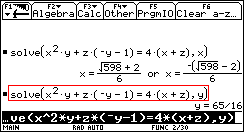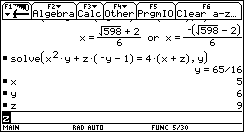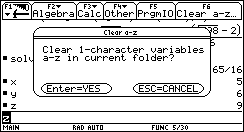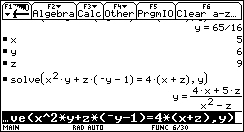### Intro to Solver on the TI-89 and TI-92

This page demonstrates first a problem and then a solution for Example 4 on page 100 of the Precalculus textbook. For that example, the textbook shows a screen image from a TI-89 to illustrate using the "solve" command to do the problem,
Solve for y: x2y + z( – y – 1) = 4 ( x + z )
Note that the screen image does not give the entire command. In order to provide a better screen image, this page will do the same problem on a TI-92. The syntax and operation of the "solve" command is the same on the two machines, but the TI-92 provides a slightly wider screen.
 Figure 1Figure 1 has been augmented to show the complete "solve" command by enclosing that command in a red box. Notice that the command has the form solve( equation, variable ) where equation holds the equation that we want to solve, and variable is the single variable whose value we seek. Unfortunately, in Figure 1, the calculator has determined that solving x2y + z( – y – 1) = 4 ( x + z ) for y gives the value 65/16. Something seems to be wrong.
Figure 1 illustrates an extremely common problem with the powerful TI-89 and TI-92 calculators. In particular, those calculators will solve problems as a string of "symbols" unless those symbols have values assigned to them. If the symbols, such as the variables x, y, and z in Figure 1, have values, then those values will be substituted for the symbols, except for the value of the variable in the solve command. It turns out that the calculator used to generate Figure 1 had, at some earlier time, assigned values to all three variables, x, y, and z. Look at Figure 2 for a confirmation of this.
 Figure 2Figure 2 shows the values that had been assigned, at some earlier time, to the variables x, y, and z, namely, x holds the value 5, y holds the value 6, and z holds the value 9. The values for x and z would have been substituted into the equation by the "solve" command, and then the value of y could be determined from the resulting values. Note that the value assigned to y did not affect the answer to the solve( x^2*y+z*( – y–1)=4(x+z),y) command. If we want to solve the original problem as it was done in the textbook, we need to clear those variables. Figure 3We can clear those values by pressing the F6 key on the TI-92 to clear all single letter variables. The calculator responds by asking for a confirmation, as is shown in Figure 3. We complete this process by pressing the ENTER key. Figure 4In Figure 4 the "Clear a-z" command has been performed, and we recalled and performed the solve( x^2*y+z*( – y–1)=4(x+z),y) command. The result as shown in Figure 4 is identical to that shown in the textbook (except that this screen is wider).